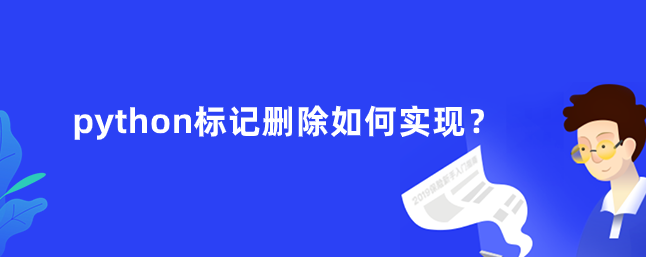## python标记如何删除

153次阅读### python标记说明

python标记主要解决循环引用的数据。因为引用大于0，不会回收。

python标记删除是为了解决这个循环引用不能删除的问题。

### python标记删除实例

```num = ["hello", "world"]
num1 = ["hi", "are", "you"]
print(sys.getrefcount(num))
print(sys.getrefcount(num1))
num.append(num1)
num1.append(num)
print(sys.getrefcount(num))
print(sys.getrefcount(num1))
del num
del num1```